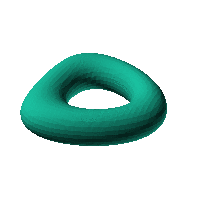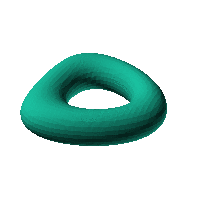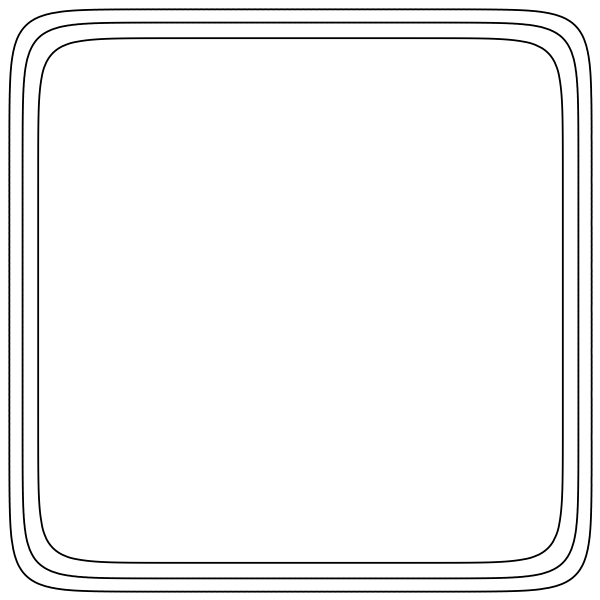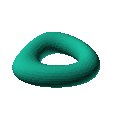Blob Farm# blob13The blob in all its glory:This is a distorted torus. It would make a good start for a torus. If you filled in the hole with something interesting, it would make a good case.

 Click on the snapshot to download the blob's stl file.Octave Code:
```From Mathematica:

f7[{x_, y_, z_}] := 1./Sqrt[(Sqrt[x^2 + y^2] - 3)^2 + z^2];

warp[{x_, y_, z_}] := {x + 0.1*y^2, y, z};
r4 = RegionPlot3D[
f7[warp[{x, -y, z}]] > 0.85, {x, -6, 6}, {y, -6, 6}, {z, -6, 6},
ImageSize -> Large, PlotPoints -> 100]
```
GNU Octave